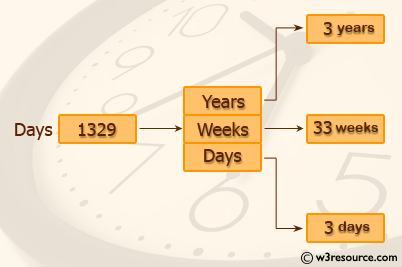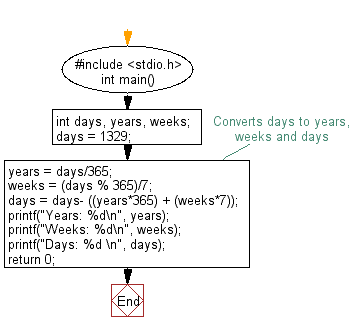﻿ C Program: Convert specified days into years, weeks and days - w3resource

# C Exercises: Convert specified days into years, weeks and days

## C Basic Declarations and Expressions: Exercise-8 with Solution

Write a C program to convert specified days into years, weeks and days.

Pictorial Presentation:Note: Ignore leap year.
Test Data :
Number of days : 1329

C Code:

``````#include <stdio.h>

int main()
{
int days, years, weeks;

days = 1329; // Total number of days

// Converts days to years, weeks and days
years = days/365; // Calculate years
weeks = (days % 365)/7; // Calculate weeks
days = days - ((years*365) + (weeks*7)); // Calculate remaining days

// Print the results
printf("Years: %d\n", years);
printf("Weeks: %d\n", weeks);
printf("Days: %d \n", days);

return 0;
}
``````

Explanation:

In the exercise above -

• The program includes the standard input/output library <stdio.h>.
• It declares three integer variables:
• int days: This variable stores the total number of days to be converted.
• int years: It stores the calculated number of years.
• int weeks: It stores the calculated number of weeks.
• The program initializes the 'days' variable with 1329.
• It then converts:
• years = days / 365: This division calculates the number of years in the given number of days. Since there are approximately 365 days in a year, this division gives an estimate of the number of years.
• weeks = (days % 365) / 7: After calculating the years, the program uses the modulo operator % to find the remaining days that couldn't be represented in full years. It then divides this remainder by 7 to calculate the number of weeks.
• Finally, it subtracts the days accounted for by years and weeks to get the remaining days.
• The program uses printf statements to display the calculated values:
• It prints the number of years with the message: "Years: [years]."
• It prints the number of weeks with the message: "Weeks: [weeks]."
• It prints the remaining days with the message: "Days: [days]."
• The program returns 0 to indicate successful execution.

Sample Output:

```Years: 3
Weeks: 33
Days: 3
```

Flowchart:C Programming Code Editor:

What is the difficulty level of this exercise?

Test your Programming skills with w3resource's quiz.

﻿